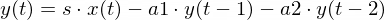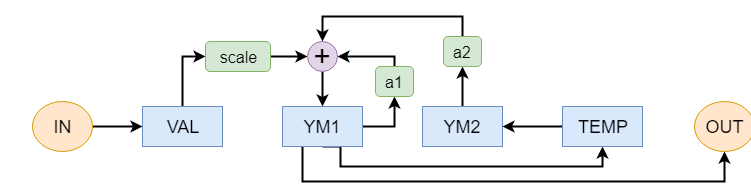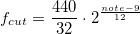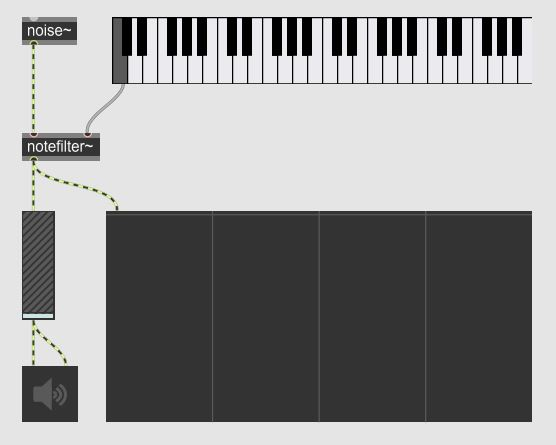# Creating an object with Max SDK

Realization of a filter for Cycling Max through Max sdk in C language.

25/05/2019 -  The Cycling Software Development Kit is a library that allows, once added among the packages, to program a new object for Max. I have read the official documentation and have done some tests; this article is the summary of what I concluded.

The idea is to create an object in C language that filters a white noise (or even an alternative noise) around a specific note, obtaining a sound as in tune as possible.
Starting from the mathematical model of a low pass filter, I have defined the simplest formulation that allowed to isolate a fairly narrow band of frequencies and with a fairly clean cut: it is of a low pass filter with an important resonance on the cutoff frequency, which I will obtain through the following formula:where s, a1 and a2 are coefficients to be calculated each time, based on even at the required cut-off frequency.

The object will be called notefilter~, and the advice to start writing its behavior in C is to clone the folder of one of the audio object examples (Max/Packages/max-sdk/source/audio) and clear the .c file, renaming appropriately all files it contains. In this way you quickly obtain a project already structured with libraries in their place. Personally I worked with Max 8, using Visual Studio in its free version. If you are using (as it is likely to be) 64-bit Max, I remind you to select that mode in the Visual Studio drop-down menu!
Here's how I wrote the notefilter.c file, step by step.

I have included the libraries necessary for the functioning of an audio object, and I have defined the object class, calling it notefilter_class
#include "ext.h"
#include "ext_obex.h"
#include "z_dsp.h"
#include <math.h>

void *notefilter_class;
specificandone poi i parametri, il cui primo (t_pxobject l_obj) è indispensabile per la corretta definizione dell'oggetto
typedef struct _notefilter
{
t_pxobject l_obj;
double freq_cut; // cutoff frequency
double l_a1; // coefficient 1
double l_a2; // coefficient 2
double l_a1p; // previous coefficient 1
double l_a2p; // previous coefficient 2
double l_ym1; // previous output sample
double l_ym2; // previous output sample
double l_fqterm; // frequency coefficient
double l_resterm; // coefficient of resonance
double l_2pidsr; // 2 pi
short l_fcon; // signal related to the frequency input
} t_notefilter;
Finally I have declared all the headers of the functions that I am going to implement, whether they are actually methods of the object class or not.
void notefilter_dsp64(t_notefilter *x, t_object *dsp64, short *count, double samplerate, long maxvectorsize, long flags);
void notefilter_perform64(t_notefilter *x, t_object *dsp64, double **ins, long numins, double **outs, long numouts, long sampleframes, long flags, void *userparam);
void notefilter_int(t_notefilter *x, int f);
void notefilter_calc(t_notefilter *x);
void *notefilter_new(double freq, double reso);
At this point the implementation of the actual functions begins, the first of which is called ext_main and takes care of physically creating the object through class_new, specifying the name, the methods of construction and destruction and the size (and other parameters that do not come used because unnecessary or obsolete). The same function also takes care of adding the methods to the class: the functions that until now are independent become methods via class_addmethod, which also requires you to pass a name to recognize that method within the function.
class_register finally allows you to register the class defined in the ClassBox of Max.
void ext_main(void *r)
{
t_class *c;
c = class_new("notefilter~",(method)notefilter_new, (method)dsp_free,
sizeof(t_notefilter), 0L, A_DEFFLOAT, A_DEFFLOAT, 0);
class_dspinit(c);
class_register(CLASS_BOX, c);
notefilter_class = c;
return 0;
}
The next function, notefilter_dsp64 , allows you to store the previous coefficients in special variables, and to store the input value provided at that instant; this step is added to the dsp chain , the list that specifies the signal processing functions defined by the object for its signals.
void notefilter_dsp64(t_notefilter *x, t_object *dsp64, short *count, double samplerate, long maxvectorsize, long flags)
{
x->l_2pidsr = (2.0 * PI) / samplerate;
notefilter_calc(x);
x->l_a1p = x->l_a1;
x->l_a2p = x->l_a2;
x->l_fcon = count;

dsp_add64(dsp64, (t_object *)x, (t_perfroutine64)notefilter_perform64, 0, NULL);
}
The core of the program lies in the next function, notefilter_perform64, which requires perhaps a slightly more detailed analysis.
After the definition of the temporary variables that are needed in the execution of the function, values ar loaded (in these newly created spaces) from the parameter set of the object, and a value called scale, given by the sum of the two coefficients increased of one is defined.
void notefilter_perform64(t_notefilter *x, t_object *dsp64, double **ins, long numins, double **outs, long numouts, long sampleframes, long flags, void *userparam) {
t_double *in = ins;
t_double *out = outs;
t_double freq = x->freq_taglio;
double a1 = x->l_a1;
double a2 = x->l_a2;
double ym1 = x->l_ym1;
double ym2 = x->l_ym2;
double val, scale, temp, resterm;

scale = 1.0 + a1 + a2;
The software now goes through all the available samples, processing the quantized value according to the mathematical formulation I have chosen.
To implement its operation, I used the temporary variables defined at the beginning of the function, and I structured a while loop to loop through the samples.
To make this key step easier to understand, I have summarized the operation in a diagram.while (sampleframes--) {
val = *in++;
temp = ym1;
ym1 = scale * val - a1 * ym1 - a2 * ym2;
ym2 = temp;
*out++ = ym1;
}
x->l_ym1 = ym1;
x->l_ym2 = ym2;
}
In Max's objects there can be different inputs, which accept data of different types. In this case, the first input will be reserved for the signal to be filtered (noise, if you want use notefilter as a synthesizer), while the second will receive an integer relative to the note midi around whose frequency you want to filter.
The notefilter_int function is in charge of defining the behavior of the object upon receiving that integer.

The cutoff frequency is calculated starting from the midi-note, following the simple formulaand its data is stored in the cut_freq parameter.
A post function (syntax analogous to the common printf function of C) allows you to print in the max console a message containing the note and frequency information in question. The function finally calls notefilter_calc, a method that performs the calculation of the coefficients, which I will discuss shortly.
void notefilter_int(t_notefilter *x, int f)
{
x->freq_taglio = (440.0 / 32.0) * pow(2.0,((f - 9.0) / 12.0));
post("nota n. %i - frequenza: %f",f, x->freq_taglio);
notefilter_calc(x);
}
The coefficients a1 and a2 are fundamental for a correct filtering operation: they characterize the intensity of the resonance and report the cutoff frequency; their calculation takes place in the function notefilter_calc according to the following expressions:and the code with which I implement it is very simple
void notefilter_calc(t_notefilter *x)
{
double resterm;
resterm = exp(0.125) * 0.882497;
x->l_fqterm = cos(x->l_2pidsr * x->freq_taglio);
x->l_a1 = -2. * resterm * x->l_fqterm;
x->l_a2 = resterm * resterm;
x->l_resterm = resterm;
}
The last function of the software, which concludes the realization of the object, is notefilter_new , what is called when creating the object.
Some routine operations are performed, in order to allocate the memory necessary for the operation of the object, and the inlets (in our case 2) and the outlets are defined (in our case 1).
Some parametric values (such as 2pidsr) can be calculated here, since their value does not have to be recalculated during the execution: these operations, in fact, come performed only when the object is created.
void *notefilter_new(double val, double reso)
{
t_notefilter *x = object_alloc(notefilter_class);
dsp_setup((t_pxobject *)x,2);

x->freq_taglio = val;
x->l_2pidsr = (2. * PI) / sys_getsr();
notefilter_calc(x);

x->l_a1p = x->l_a1;
x->l_a2p = x->l_a2;

outlet_new((t_object *)x, "signal");
return (x);
}

The software works, and once compiled it has successfully generated the notefilter~ object, which appears now among Max's objects, complete with auto-fill when you start typing its name.
To test this, I made a simple patch where the noise produced by a noise~ object is filtered from notefilter~, which receives from a kslider the note around which to filter the signal. The output is viewed via the spectroscope and reproduced via the sound card via the object ezdac.The result is a metallic sound and definitely not suitable for real use, but I am satisfied with how much I got because, after all, that's what I expected. This is what the spectroscope shows during notefilter operationThe complete notefilter.c file is available at the following link: